### Thermomechanical iterations

To find the solution at the end of a given increment a set of nonlinear equations has to be solved. In order to do so, the Newton-Raphson method is used, i.e. the set of equations is locally linearized and solved. If the solution does not satisfy the original nonlinear equations, the latter are again linearized at the new solution. This procedure is repeated until the solution satisfies the original nonlinear equations within a certain margin. Suppose iteration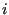has been performed and convergence is to be checked. Let us introduce the following quantities:

•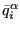: the average flux for field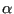at the end of iteration. It is defined by: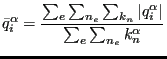(592)

where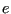represents all elements,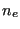all nodes belonging to a given element,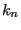all degrees of freedom for fieldbelonging to a given node and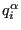is the flux for a given degree of freedom of fieldin a given node belonging to a given element at the end of iteration. Right now, there are two kind of fluxes in CalculiX: the force for mechanical calculations and the concentrated heat flux for thermal calculations.

•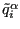: the iteration-average of the average flux for fieldof all iterations in the present increment up to but not including iteration.

•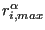: the largest residual flux (in absolute value) of fieldat the end of iteration. For its calculation each degree of freedom is considered independently from all others: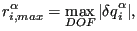(593)

where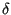denotes the change due to iteration.

•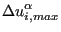: the largest change in solution (in absolute value) of fieldin the present increment, i.e. the solution at the end of iteration i of the present increment minus the solution at the start of the increment :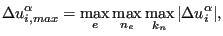(594)

where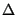denotes the change due to the present increment. In mechanical calculations the solution is the displacement, in thermal calculations it is the temperature.

•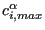: the largest change in solution (in absolute value) of fieldin iteration. :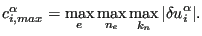(595)

Now, two constants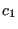and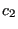are introduced:is used to check convergence of the flux,serves to check convergence of the solution. Their values depend on whether zero flux conditions prevail or not. Zero flux is defined by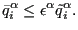(596)

The following rules apply:

• if(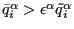) (no zero flux):

• if (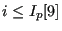)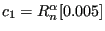,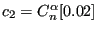.
• else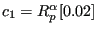,.

• else (zero flux)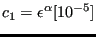,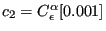The values in square brackets are the default values. They can be changed by using the keyword card *CONTROLS. Now, convergence is obtained if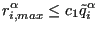(597)

AND if, for thermal or thermomechanical calculations (*HEAT TRANSFER, *COUPLED TEMPERATURE-DISPLACEMENT or *UNCOUPLED TEMPERATURE-DISPLACEMENT), the temperature change does not exceed DELTMX,

AND at least one of the following conditions is satisfied:

•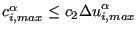•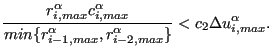(598)

The left hands side is an estimate of the largest solution correction in the next iteration. This condition only applies if no gas temperatures are to be calculated (no forced convection).
•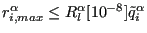. If this condition is satisfied, the increment is assumed to be linear and no solution convergence check is performed. This condition only applies if no gas temperatures are to be calculated (no forced convection).
•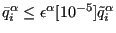(zero flux conditions). This condition only applies if no gas temperatures are to be calculated (no forced convection).
•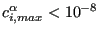.

If convergence is reached, and the size of the increments is not fixed by the user (no parameter DIRECT on the *STATIC, *DYNAMIC or *HEAT TRANSFER card) the size of the next increment is changed under certain circumstances:

• if(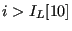):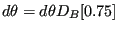, where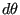is the increment size relative to the step size (convergence was rather slow and the increment size is decreased).
• if(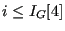) AND the same applies for the previous increment: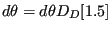(convergence is fast and the increment size is increased).

If no convergence is reached in iteration, the following actions are taken:

• if, for thermomechanical calculations, the temperature change exceeds DELTMX, the size of the increment is multiplied by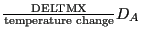[0.85].
• if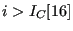, too many iterations are needed to reach convergence and any further effort is abandoned: CalculiX stops with an error message.
• if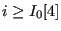AND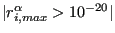AND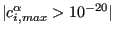AND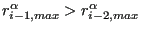AND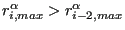AND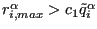then:
• if the parameter DIRECT is active, the solution is considered to be divergent and CalculiX stops with an error message.
• else, the size of the increment is adapted according to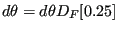and the iteration of the increment is restarted.
• if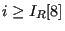, the number of iterations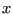is estimated needed to reach convergence.roughly satisfies: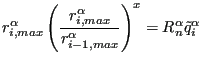(599)

from whichcan be determined. Now, if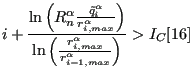(600)

(which means that the estimated number of iterations needed to reach convergence exceeds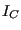) OR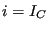, the increment size is adapted according to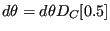and the iteration of the increment is restarted unless the parameter DIRECT was selected. In the latter case the increment is not restarted and the iterations continue.

• if none of the above applies iteration continues.